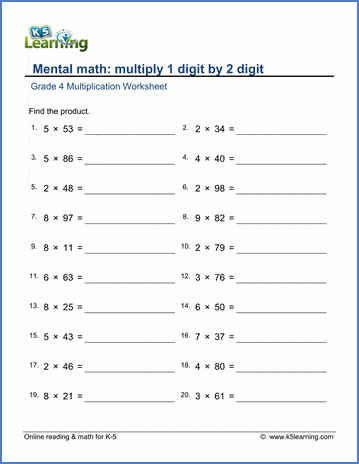# Multiplication Worksheets

## Multiplication worksheets for grades 2 to 6

Our multiplication worksheets start with the basic multiplication facts and progress to multiplying large numbers in columns.  We emphasize "mental multiplication" exercises to improve numeracy skills.

Grade 4 multiply in columns worksheets

Multi-digit multiplication: drills and practice

Multiplication flashcards

Topics include:

• Meaning of multiplication
• Arrays
• Multiplication Facts 2 & 3, 5, 10, 2-5
• Multiplication Tables of 2, 5 & 10
• Multiplication tables - missing factors
• Two times small numbers
• Two times multiples of 5
• Two times whole tens
• Two times whole tens (missing factors)
• Multiplication word problems (within 25)

• Meaning of multiplication
• Multiplication sentences
• Multiply with arrays
• Multiply using a number line
• Multiplication facts (various practice up to 2-12)
• Multiplication tables
• Multiplication facts (missing factors)
• Multiplying 1-digit numbers by whole tens
• Multiplying 1-digit numbers by whole hundreds
• Multiply whole tens by whole tens
• Multiply whole tens (missing factors)
• Multiply in columns (1-digit by 2-4 digits)
• Multiplication word problems

## Grade 4 mental multiplication worksheets

• Multiplication tables 2-10, 2-12, random facts
• Multiplication tables 2-10, 2-12, missing factors
• Commutative property
• Distributive property
• Multiply 1-dit numbers by whole tens or hundreds
• Multiplying whole tens by whole tens (including missing factors)
• Multiply whole tens, whole hundreds and whole thousands
• Multiply 1-digit numbers by a number close to 100
• Multiply in parts (1-digit by 2 or 3 digits)
• Mixed multiplication and division word problems
• Mixed 4 operations word problems

## Grade 4 multiplication in columns worksheets

• Multiply in columns 1-digit by 2, 3 or 4 digits
• Multiply in columns 2-digits by 2, 3 or 4 digits
• Multiply in columns 3 digit by 3 digit

• Multiply by 10, 100 or 1,000 with missing factors
• Multiplying in parts (distributive property)
• Multiply 1 digit by 3 digit numbers mentally
• Multiply in columns up to 2x4 digits and 3x3 digits
• Mixed 4 operations word problems

• Distributive property
• Multiplying in columns up to 5 digit numbers

## Related topics

Division worksheets

Fractions worksheetsSample Multiplication Worksheet

What is K5?

K5 Learning offers free worksheets, flashcards and inexpensive workbooks for kids in kindergarten to grade 5. Become a member to access additional content and skip ads.